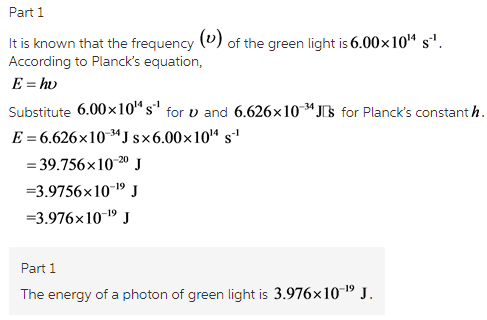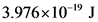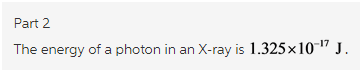# Green light has a frequency of about 6.00 x 10^14 s-1 . What is the energy of a photon of green light?

Green light has a frequency of about 6.00 x 10^14 s-1 . What is the energy of a photon of green light?
4 sigfig
E=J

Hospital X-ray generators emit X rays with wavelength of about 15.0 nanometers (), where 1 nm = 10^9 m. What is the energy of a photon in an X ray?

Concepts and reason
The concept used to solve this problem is based on the energy of a photon.
The energy of the photons is calculated using the Planck’s law when their frequency is known. The energy can also be calculated when only the wavelength is known. The wave equation is used to first calculate the frequency and then it is substituted in the Planck’s equation.

Fundamentals
The energy of a photon can be calculated using the Planck’s equation:Since the answer has to be reported in 4 significant figures, therefore the energy of a photon of green light is